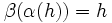# Retract is transitive

This article gives the statement, and possibly proof, of a subgroup property (i.e., retract) satisfying a subgroup metaproperty (i.e., transitive subgroup property)
View all subgroup metaproperty satisfactions | View all subgroup metaproperty dissatisfactions |Get help on looking up metaproperty (dis)satisfactions for subgroup properties
Get more facts about retract |Get facts that use property satisfaction of retract | Get facts that use property satisfaction of retract|Get more facts about transitive subgroup property

## Statement

Suppose$H \le G \le K$ are groups such that$G$ is a retract of$K$ and$H$ is a retract of$G$, then$H$ is a retract of$K$.

## Definitions used

### Retract

Further information: Retract

A subgroup$H$ of a group$G$ is termed a retract of$G$ if there exists a homomorphism$\sigma:G \to H$ such that$\sigma(h) = h$ for all$h \in H$.

## Proof

Given: A group$K$, a retract$G$ of$K$, a retract$H$ of$G$.

To prove:$H$ is a retract of$K$.

Proof: Let$\alpha:K \to G$ be a retraction, i.e.,$\alpha$ is a homomorphism such that$\alpha(g) = g$ for all$g \in G$. Let$\beta:G \to H$ be a retraction, i.e.,$\beta$ is a homomorphism such that$\beta(h) = h$ for all$h \in H$.

Now consider the composite map$\beta \circ \alpha: K \to H$. We want to argue that this is a retraction. Consider$h \in H$. Then, by construction$\alpha(h) = h$, so$\beta(\alpha(h)) = \beta(h)$. Since$H \le G$,$\beta(h) = h$, and so we get$\beta(\alpha(h)) = h$. Thus,$\beta \circ \alpha$ is a retraction, and thus$H$ is a retract of$K$.